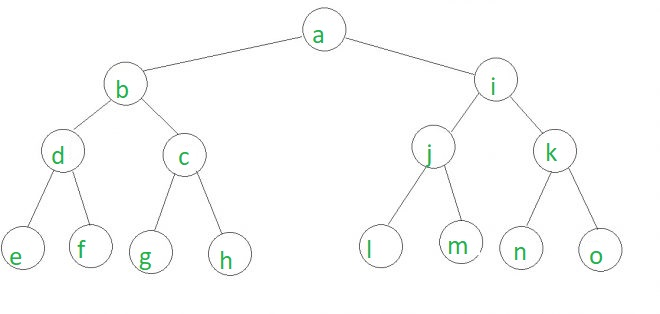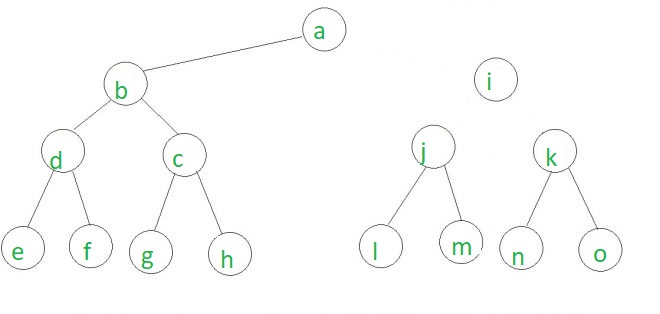# Remove edges connected to a node such that the three given nodes are in different trees

Given a binary tree and 3 nodes a, b and c, the task is to find a node in the tree such that after removing all the edge connected to that node, a, b and c are in three different trees.

Given below is a tree with input nodes as c, j and o.In the above tree, if node i gets disconnected from the tree, then the given nodes c, j, and o will be in three different trees which have been shown below.## Recommended: Please try your approach on {IDE} first, before moving on to the solution.

A simple approach is to find LCA of all possible pairs of nodes given.
Let,

• lca of ( a, b) = x
• lca of (b, c) = y
• lca of (c, a) = z

In any case, either of (x, y), (y, z), (z, x) or (x, y, z) will always be the same. In the first three cases, return the node which is not the same. In the last case returning any node of x, y or z will give the answer.

Below is the implementation of the above approach:

## C++

 `// C++ program for disconnecting a ` `// node to result in three different tree ` `#include ` `using` `namespace` `std; ` ` `  `// node class ` `struct` `Node { ` `    ``int` `key; ` `    ``struct` `Node *left, *right; ` `}; ` `Node* newNode(``int` `key) ` `{ ` `    ``Node* temp = ``new` `Node; ` `    ``temp->key = key; ` `    ``temp->left = temp->right = NULL; ` `    ``return` `(temp); ` `} ` ` `  `// LCA function taken from the above link mentioned ` `// This function returns a pointer to LCA of two given ` `// values n1 and n2. This function assumes that n1 and n2 ` `// are present in Binary Tree ` `struct` `Node* findLCA(``struct` `Node* root, ``int` `n1, ``int` `n2) ` `{ ` `    ``// Base case ` `    ``if` `(root == NULL) ` `        ``return` `NULL; ` ` `  `    ``// If either n1 or n2 matches with root's key, report ` `    ``// the presence by returning root (Note that if a key is ` `    ``// ancestor of other, then the ancestor key becomes LCA ` `    ``if` `(root->key == n1 || root->key == n2) ` `        ``return` `root; ` ` `  `    ``// Look for keys in left and right subtrees ` `    ``Node* left_lca = findLCA(root->left, n1, n2); ` `    ``Node* right_lca = findLCA(root->right, n1, n2); ` ` `  `    ``// If both of the above calls return Non-NULL, then one key ` `    ``// is present in once subtree and other is present in other, ` `    ``// So this node is the LCA ` `    ``if` `(left_lca && right_lca) ` `        ``return` `root; ` ` `  `    ``// Otherwise check if left subtree or right subtree is LCA ` `    ``return` `(left_lca != NULL) ? left_lca : right_lca; ` `} ` ` `  `// the function assumes a, b, c are present in the tree ` `// and returns a node disconnecting which ` `// results in all three nodes in different trees ` `Node* findNode(Node* root, ``int` `a, ``int` `b, ``int` `c) ` `{ ` `    ``// lca of a, b ` `    ``Node* x = findLCA(root, a, b); ` ` `  `    ``// lca of b, c ` `    ``Node* y = findLCA(root, b, c); ` ` `  `    ``// lca of c, a ` `    ``Node* z = findLCA(root, c, a); ` ` `  `    ``if` `(x->key == y->key) ` `        ``return` `z; ` `    ``else` `if` `(x->key == z->key) ` `        ``return` `y; ` `    ``else` `        ``return` `x; ` `} ` ` `  `// Driver Code ` `int` `main() ` `{ ` `    ``// Declare tree ` `    ``// Insert elements in the tree ` `    ``Node* root = newNode(1); ` ` `  `    ``root->left = newNode(2); ` `    ``root->right = newNode(3); ` ` `  `    ``root->left->left = newNode(4); ` `    ``root->left->right = newNode(5); ` ` `  `    ``root->left->left->left = newNode(8); ` `    ``root->left->left->right = newNode(9); ` ` `  `    ``root->left->right->left = newNode(10); ` `    ``root->left->right->right = newNode(11); ` ` `  `    ``root->right->left = newNode(6); ` `    ``root->right->right = newNode(7); ` `    ``root->right->left->left = newNode(12); ` `    ``root->right->left->right = newNode(13); ` `    ``root->right->right->left = newNode(14); ` `    ``root->right->right->right = newNode(15); ` ` `  `    ``/* ` `            ``1 ` `        ``/     \ ` `       ``2       3 ` `     ``/  \     /  \ ` `    ``4   5     6    7 ` `   ``/\  / \   / \  / \ ` `  ``8 9 10 11 12 13 14 15      ` `                           ``*/` ` `  `    ``// update all the suitable_children ` `    ``// keys of all the nodes in O( N ) ` ` `  `    ``cout << ``"Disconnect node "` `         ``<< findNode(root, 5, 6, 15)->key ` `         ``<< ``" from the tree"``; ` ` `  `    ``return` `0; ` `} `

## Java

 `// Java program for disconnecting a  ` `// node to result in three different tree  ` `public` `class` `RemoveEdge { ` ` `  `    ``// LCA function taken from the above link mentioned  ` `    ``// This function returns a pointer to LCA of two given  ` `    ``// values n1 and n2. This function assumes that n1 and n2  ` `    ``// are present in Binary Tree  ` `    ``static` `Node findLCA(Node root, ``int` `n1, ``int` `n2)  ` `    ``{  ` `        ``// Base case  ` `        ``if` `(root == ``null``)  ` `            ``return` `root;  ` `   `  `        ``// If either n1 or n2 matches with root's key, report  ` `        ``// the presence by returning root (Note that if a key is  ` `        ``// ancestor of other, then the ancestor key becomes LCA  ` `        ``if` `(root.key == n1 || root.key == n2)  ` `            ``return` `root;  ` `   `  `        ``// Look for keys in left and right subtrees  ` `        ``Node left_lca = findLCA(root.left, n1, n2);  ` `        ``Node right_lca = findLCA(root.right, n1, n2);  ` `   `  `        ``// If both of the above calls return Non-NULL, then one key  ` `        ``// is present in once subtree and other is present in other,  ` `        ``// So this node is the LCA  ` `        ``if` `(left_lca!=``null` `&& right_lca!=``null``)  ` `            ``return` `root;  ` `   `  `        ``// Otherwise check if left subtree or right subtree is LCA  ` `        ``return` `(left_lca != ``null``) ? left_lca : right_lca;  ` `    ``}  ` `   `  `    ``// the function assumes a, b, c are present in the tree  ` `    ``// and returns a node disconnecting which  ` `    ``// results in all three nodes in different trees  ` `    ``static` `Node findNode(Node root, ``int` `a, ``int` `b, ``int` `c)  ` `    ``{  ` `        ``// lca of a, b  ` `        ``Node x = findLCA(root, a, b);  ` `        ``// lca of b, c  ` `        ``Node y = findLCA(root, b, c);  ` `        ``// lca of c, a  ` `        ``Node z = findLCA(root, c, a);  ` `   `  `        ``if` `(x.key == y.key)  ` `            ``return` `z;  ` `        ``else` `if` `(x.key == z.key)  ` `            ``return` `y;  ` `        ``else` `            ``return` `x;  ` `    ``}  ` ` `  `    ``public` `static` `void` `main(String args[]) { ` `        ``Node root = ``new` `Node(``1``);  ` `        ``root.left = ``new` `Node(``2``);  ` `        ``root.right = ``new` `Node(``3``);  ` `        ``root.left.left = ``new` `Node(``4``);  ` `        ``root.left.right = ``new` `Node(``5``);  ` `        ``root.left.left.left = ``new` `Node(``8``);  ` `        ``root.left.left.right = ``new` `Node(``9``);  ` `        ``root.left.right.left = ``new` `Node(``10``);  ` `        ``root.left.right.right = ``new` `Node(``11``);  ` `        ``root.right.left = ``new` `Node(``6``);  ` `        ``root.right.right = ``new` `Node(``7``);  ` `        ``root.right.left.left = ``new` `Node(``12``);  ` `        ``root.right.left.right = ``new` `Node(``13``);  ` `        ``root.right.right.left = ``new` `Node(``14``);  ` `        ``root.right.right.right = ``new` `Node(``15``);  ` `        ``System.out.print(``"Disconnect node "``+findNode(root, ``5``, ``6``, ``15``).key+``" from the tree"``); ` `    ``} ` `} ` ` `  `// Node class  ` `class` `Node {  ` `    ``int` `key;  ` `    ``Node left, right;  ` `    ``Node (``int` `data) ` `    ``{ ` `        ``this``.key=data; ` `    ``} ` `};  ` `//This code is contributed by Gaurav Tiwari `

## C#

 `// C# program for disconnecting a  ` `// node to result in three different tree  ` `using` `System; ` ` `  `public` `class` `RemoveEdge ` `{  ` ` `  `    ``// LCA function taken from the  ` `    ``// above link mentioned This function ` `    ``// returns a pointer to LCA of two given  ` `    ``// values n1 and n2. This function ` `    ``// assumes that n1 and n2  ` `    ``// are present in Binary Tree  ` `    ``static` `Node findLCA(Node root, ``int` `n1, ``int` `n2)  ` `    ``{  ` `        ``// Base case  ` `        ``if` `(root == ``null``)  ` `            ``return` `root;  ` `     `  `        ``// If either n1 or n2 matches  ` `        ``// with root's key, report  ` `        ``// the presence by returning  ` `        ``// root (Note that if a key is  ` `        ``// ancestor of other, then the  ` `        ``// ancestor key becomes LCA  ` `        ``if` `(root.key == n1 || root.key == n2)  ` `            ``return` `root;  ` `     `  `        ``// Look for keys in left and right subtrees  ` `        ``Node left_lca = findLCA(root.left, n1, n2);  ` `        ``Node right_lca = findLCA(root.right, n1, n2);  ` `     `  `        ``// If both of the above calls  ` `        ``// return Non-NULL, then one key  ` `        ``// is present in once subtree and  ` `        ``// other is present in other,  ` `        ``// So this node is the LCA  ` `        ``if` `(left_lca!=``null` `&& right_lca!=``null``)  ` `            ``return` `root;  ` `     `  `        ``// Otherwise check if left  ` `        ``// subtree or right subtree is LCA  ` `        ``return` `(left_lca != ``null``) ? left_lca : right_lca;  ` `    ``}  ` `     `  `    ``// the function assumes a, b, c  ` `    ``// are present in the tree and returns ` `    ``// a node disconnecting which results ` `    ``// in all three nodes in different trees  ` `    ``static` `Node findNode(Node root, ``int` `a, ``int` `b, ``int` `c)  ` `    ``{  ` `        ``// lca of a, b  ` `        ``Node x = findLCA(root, a, b);  ` `         `  `        ``// lca of b, c  ` `        ``Node y = findLCA(root, b, c);  ` `         `  `        ``// lca of c, a  ` `        ``Node z = findLCA(root, c, a);  ` `     `  `        ``if` `(x.key == y.key)  ` `            ``return` `z;  ` `        ``else` `if` `(x.key == z.key)  ` `            ``return` `y;  ` `        ``else` `            ``return` `x;  ` `    ``}  ` ` `  `    ``// Driver code ` `    ``public` `static` `void` `Main(String []args)  ` `    ``{  ` `        ``Node root = ``new` `Node(1);  ` `        ``root.left = ``new` `Node(2);  ` `        ``root.right = ``new` `Node(3);  ` `        ``root.left.left = ``new` `Node(4);  ` `        ``root.left.right = ``new` `Node(5);  ` `        ``root.left.left.left = ``new` `Node(8);  ` `        ``root.left.left.right = ``new` `Node(9);  ` `        ``root.left.right.left = ``new` `Node(10);  ` `        ``root.left.right.right = ``new` `Node(11);  ` `        ``root.right.left = ``new` `Node(6);  ` `        ``root.right.right = ``new` `Node(7);  ` `        ``root.right.left.left = ``new` `Node(12);  ` `        ``root.right.left.right = ``new` `Node(13);  ` `        ``root.right.right.left = ``new` `Node(14);  ` `        ``root.right.right.right = ``new` `Node(15);  ` `        ``Console.Write(``"Disconnect node "``+ ` `                        ``findNode(root, 5, 6, 15).key+ ` `                        ``" from the tree"``);  ` `    ``}  ` `}  ` ` `  `// Node class  ` `public` `class` `Node  ` `{  ` `    ``public` `int` `key;  ` `    ``public` `Node left, right;  ` `    ``public` `Node (``int` `data)  ` `    ``{  ` `        ``this``.key=data;  ` `    ``}  ` `};  ` ` `  `// This code contributed by Rajput-Ji `

Output:

```Disconnect node 3 from the tree
```

My Personal Notes arrow_drop_upCheck out this Author's contributed articles.

If you like GeeksforGeeks and would like to contribute, you can also write an article using contribute.geeksforgeeks.org or mail your article to contribute@geeksforgeeks.org. See your article appearing on the GeeksforGeeks main page and help other Geeks.

Please Improve this article if you find anything incorrect by clicking on the "Improve Article" button below.

Improved By : _Gaurav_Tiwari, Rajput-Ji

Article Tags :
Practice Tags :

1

Please write to us at contribute@geeksforgeeks.org to report any issue with the above content.